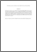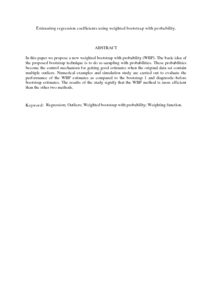# Estimating regression coefficients using weighted bootstrap with probability.

## Citation

Mohamed Ramli, Norazan and Midi, Habshah and Imon, A. H. M. R. (2009) Estimating regression coefficients using weighted bootstrap with probability. WSEAS Transactions on Mathematics, 8 (7). pp. 362-371. ISSN 1109-2769; ESSN: 2224-2880

## Abstract

In this paper we propose a new weighted bootstrap with probability (WBP). The basic idea of the proposed bootstrap technique is to do re-sampling with probabilities. These probabilities become the control mechanism for getting good estimates when the original data set contain multiple outliers. Numerical examples and simulation study are carried out to evaluate the performance of the WBP estimates as compared to the bootstrap 1 and diagnostic-before bootstrap estimates. The results of the study signify that the WBP method is more efficient than the other two methods.Preview
PDF (Abstract)
Estimating regression coefficients using weighted bootstrap with probability.pdfView Item